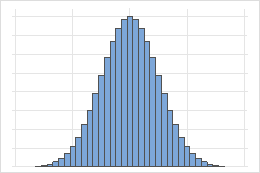# Binomial distribution

A binomial distribution is a discrete distribution that models the number of events in a fixed number of trials. Each trial has two possible outcomes and event is the outcome of interest from a trial.

Use the binomial distribution to describe a process where the outcomes can be labeled as an event or nonevent and when you are interested in the occurrence of an event and not in its magnitude. For example, an item passes or fails inspection or a political party wins or loses. The binomial distribution is frequently used in quality control, public opinion surveys, medical research, and insurance.

For example, use the binomial distribution to calculate the probability that 3 or more defectives are in a sample of 25 items if the probability of a defective for each trial is 0.02. The number of defective items (X) follows a binomial distribution with n = 25 and p = 0.02.

The number of events (X) in n trials follows a binomial distribution if the following conditions are met:
• The number of trials is fixed.
• Each trial is independent of the other trials.
• Each trial has one of two outcomes: event or nonevent.
• The probability of an event is the same for each trial.
One of the properties of a binomial distribution is that when n is large, the binomial distribution can be reasonably approximated by the normal distribution. For example, for following binomial distribution, n = 100 and p = 0.5.By using this site you agree to the use of cookies for analytics and personalized content.  Read our policy